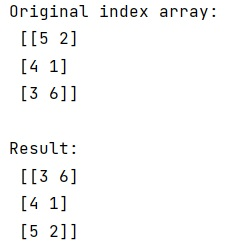# How to Sort NumPy Arrays by Column?

Learn, how to sort NumPy arrays by column in Python? By Pranit Sharma Last updated : March 28, 2023

Suppose that we are given a multi-dimensional numpy array and we need to sort data by the first column to return an array where all the values of the first columns are sorted.

## Sorting arrays in NumPy by column

To sort NumPy arrays by column in Python, we use numpy.argsort() where we will pass the sliced array part i.e, the first column of the array but this will only return the sorted indices and hence we need to pass this result in the indexing of the original array.

Let us understand with the help of an example,

## Python code to sort NumPy arrays by column

```# Import numpy
import numpy as np

# Creating index array
arr = np.array([[5,2], [4,1], [3,6]])

# Display Original array
print("Original index array:\n",arr,"\n")

# column by which we need to sort the array
col = 0

# Sorting by column
res = arr[np.argsort(arr[:,col])]

# Display result
print("Result:\n",res)
```

### Output